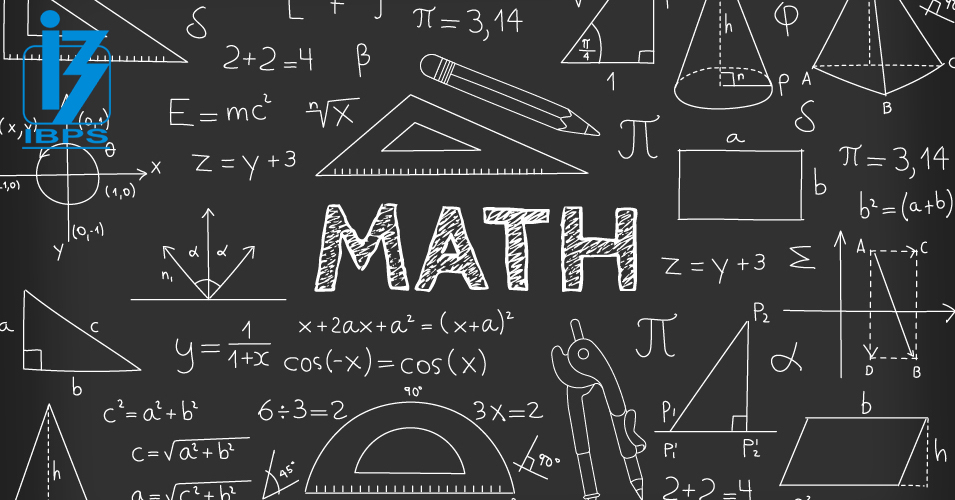• Save

# Maths Inequality Questions for Bank Po Exams

Last month 9.2K ViewsInequality topic is very important in the logical reasoning section. Here are given maths inequality questions for bank exam and other Competitive exams. In these questions, you have to solve the questions according to compare into two quantities.

You can easily understand this topic by practicing these maths inequality questions. So, continue your practice with these questions and improve your performance as well as.

## Inequality Questions of Maths for Competitive Exams

Directions: Each question below contains a statement followed by Quantity I and Quantity II. You have to study the information along with the question and compare the value derived from Quantity I and Quantity II, then answer:

Q.1. What is the age of Amanpreet?

Quantity I: Amanpreet is 3 years younger than Mohanpreet and the ratio of their ages is 7 : 8.

Quantity II: Average age of Meet and Amanpreet is 25 years and Meet is 2 years older than Amanpreet.

(A) Quantity I = Quantity II

(B) Quantity I > Quantity II

(C) Quantity I ≥ Quantity II

(D) Quantity I < Quantity II

(E) Quantity I ≤ Quantity II

Ans .   D

Q.2. Find the percentage of boys in the class this year.

Quantity I: This year the percentage of girls in the class is 60%.

Quantity II: Last years out of the 300 students, 50% was girls and this year the number of girls are increased by 10% but total students remains same.

(A)  Quantity I < Quantity II

(B) Quantity I > Quantity II

(C) Quantity I ≤ Quantity II

(D) Quantity I = Quantity II

(E) Quantity I ≥ Quantity II

Ans .   A

Q.3. What is the volume of the cylinder?

Quantity I: The curved surface area of the cylinder is 220 cm2 and the height of the cylinder is 2 cm less than the radius of the cylinder.

Quantity II: 770 cm3.

(A) Quantity I ≥ Quantity II

(B)  Quantity I < Quantity II

(C)  Quantity I ≤ Quantity II

(D) Quantity I = Quantity II

(E) Quantity I > Quantity II

Ans .   D

Q.4. If a and b are natural numbers and 7 > a > b > 3.

Quantity I: 4a3b

Quantity II: 2a2b2

(A)  Quantity I > Quantity II

(B) Quantity I = Quantity II

(C) Quantity I ≥ Quantity II

(D) Quantity I < Quantity II

(E) Quantity I ≤ Quantity II

Ans .   A

Q.5. In an envelope, there are 5 green, 3 yellow and 4 pink tablets. 3 tablets are picked at random.

Quantity I: The probability that 2 tablets are yellow in colour and 1 tablet is pink in colour.

Quantity II: The probability that all the tablets are green in colour.

(A) Quantity I < Quantity II

(B) Quantity I > Quantity II

(C) Quantity I ≥ Quantity II

(D) Quantity I = Quantity II

(E) Quantity I ≤ Quantity II

Ans .   B

Feel free and ask me in the comment section if you face any problem related to maths inequality questions. Visit on the next page for more practice.